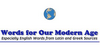DecimetreThe decimetre (International spelling as used by the International Bureau of Weights and Measures; SI symbol dm) or decimeter (American spelling) is a unit of length in the metric system, equal to one tenth of a metre, the SI base unit of length (or ten centimetres). Equivalently, there are 10 decimetres in a metre. It is equivalent to 3.93700787 ...
Found on http://en.wikipedia.org/wiki/Decimetre

Decimetre• (n.) A measure of length in the metric system; one tenth of a meter, equal to 3.937 inches.
Found on http://thinkexist.com/dictionary/meaning/decimetre/

decimetre<unit> One-tenth of a metre. ... Equivalent to 3.973 inches, 10 cm, or 0.1 m. ... (09 Oct 1997) ...
Found on http://www.encyclo.co.uk/local/20973

decimetreAbbreviated as dm, unit length equal to 10-1m. See also: Decimal Prefixes, Metre.
Found on http://www.diracdelta.co.uk/science/source/d/e/decimetre/source.html

decimetredecimetre (British)
Found on http://www.wordinfo.info/words/index/info/view_unit/1302/11
No exact match found